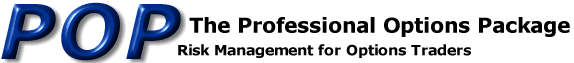Online Options Help
Pricing Inputs

Online Pricing and Analysis

Call or Put: Select one or the other.

Strike Price: Enter the strike price of the option.

Options Quantity: How many options are being analyzed. Use a negative sign to indicate that the trade is a sell.

Futures Quantity: When trading options, buying or selling the underlying can be used to offset the trade. Enter the number of hedge contracts here. Again, enter sells as a negative.CONTRACT SPECIFICATIONS

The following values allow you to customize the risk analysis for a particular commodity.

American Exercise v. European Exercise: If early exercise is permitted, prices are calculated using the quadratic approximation model by Whaley, et. al. For European exercise, P.O.P. uses a standard Black-Scholes model.

Eurodollar Style: For pricing a Eurodollar, Libor, or other certain interest rates products, special pricing methods are employed. If the price of the underlying cannot exceed 100.00 without indicating a negative interest rate, you should select this checkbox.

Futures Ticks per Point: If the underlying trades in fractions such as eighths or thirty-seconds, enter 8, 32, or any whole number here. If the underlying is decimal based, the futures ticks should be 100.

Options Ticks per Point: If the options trade in fractions, enter that number here.

Example Specifications

Risk Statistics in \$ (not ticks): If you prefer to see the position's theta, vega, and profit/loss projections in dollars, pounds, or yen rather than ticks, check this box and enter a number in the Dollars per Point box below.

Dollars per Point: If the underlying moves from 100.00 to 101.00 or from 22-03 to 23-03, how much money would you make if you were long one of those contracts.

MARKET INPUTS

These are the parameters used by the pricing models to generate theoretical values and risk statistics. You can use the observed (current) market conditions. In the case of volatility, many traders use historical levels. If you need current market values for one of the more popular contracts, you can refer to our Daily Options Summary.

Futures: The price of the underlying instrument. The difference between the price of the underlying and the strike price determines intrinsic value. Enter prices as 100.50 for decimal based contracts and 100-16 for contracts that trade in fractions such as eighths or sixteenths.

Days: The number of days remaining until expiration of the contract. A 365 day calendar year is used. That means weekends and holidays are counted.

Volatility: The volatility of the underlying. The different estimates include implied, historical, and projected.

Interest Rate: The interest rate that is used to compute the cost of carry.

 For convenience, volatilities and interest rates can be entered as percentages (use 15.5 for 15.5%) or decimals (use .155 for 15.5%). Make sure that the box is checked if you prefer tp use percentages.PROJECTION INCREMENTS

Futures Increment: This input determines the range of prices that the position's risk is projected. Increase this number to see a wider range.

Days Increment: By putting a value in this box, a second risk projection is displayed. This projection shows what your position will look like at some point in the future. If there are 20 days left in the life of the option, and you set the value of the days increment to 20, you will see the position at expiration.

Volatility Increment: Similar to the Days Increment, it is possible to see the position profiled at a volatility higher or lower than the current value.e-mail: info@pmpublishing.com